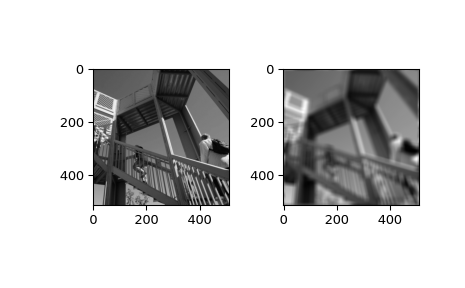# scipy.ndimage.fourier_uniform¶

scipy.ndimage.fourier_uniform(input, size, n=-1, axis=-1, output=None)[source]

Multidimensional uniform fourier filter.

The array is multiplied with the Fourier transform of a box of given size.

Parameters
inputarray_like

The input array.

sizefloat or sequence

The size of the box used for filtering. If a float, size is the same for all axes. If a sequence, size has to contain one value for each axis.

nint, optional

If n is negative (default), then the input is assumed to be the result of a complex fft. If n is larger than or equal to zero, the input is assumed to be the result of a real fft, and n gives the length of the array before transformation along the real transform direction.

axisint, optional

The axis of the real transform.

outputndarray, optional

If given, the result of filtering the input is placed in this array. None is returned in this case.

Returns
fourier_uniformndarray

The filtered input.

Examples

>>> from scipy import ndimage, misc
>>> import numpy.fft
>>> import matplotlib.pyplot as plt
>>> fig, (ax1, ax2) = plt.subplots(1, 2)
>>> plt.gray()  # show the filtered result in grayscale
>>> ascent = misc.ascent()
>>> input_ = numpy.fft.fft2(ascent)
>>> result = ndimage.fourier_uniform(input_, size=20)
>>> result = numpy.fft.ifft2(result)
>>> ax1.imshow(ascent)
>>> ax2.imshow(result.real)  # the imaginary part is an artifact
>>> plt.show()#### Previous topic

scipy.ndimage.fourier_shift

#### Next topic

scipy.ndimage.affine_transform